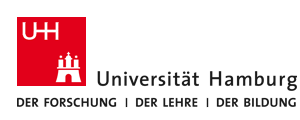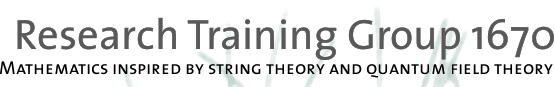# Core Research Area I: Integrable models

Since their discovery in the XIX century, integrable models continue to be a rich source of inspiration for various fields of mathematics. Early examples are the theory of hyperelliptic functions founded by Jacobi to solve the Neumann model, or the Lax pair approach, which in its modern incarnation is known as the inverse scattering method and is a powerful tool to solve a certain class of non-linear partial differential equations. Today, the theory of integrable models has a wide, steadily growing range of applications in theoretical physics. Mathematically, integrable models have stimulated and connected important branches of geometry, algebra, and analysis. There are hints towards a more unified picture: A large class of classically integrable models have phase spaces which are the moduli spaces of flat or self-dual connections, or the moduli spaces of solutions to other partial differential equations on certain manifolds. The Poisson structures on the respective phase spaces descend from natural Poisson structures on the relevant spaces of geometric structures. The quantisation of such Poisson structures often produces particular Hopf algebras known as quantum groups. A universal scheme is gradually emerging for the study of quantum integrable models based on the representation theory of quantum groups. The relevant moduli spaces represent the moduli spaces of vacua for a large class of supersymmetric gauge theories [NS]. Two-dimensional sigma models on (generalized) symmetric spaces provide another important class of integrable models which play a pivotal role in statistical physics and string theory. Over the last few years, models on supersymmetric coset spaces have received particular attention. For a few examples which are related to gauge theory via the AdS/CFT correspondence, remarkable progress has been achieved in uncovering the spectrum of such theories, see e.g. [AF], [GKLV].

An interesting family of finite-dimensional quantum-mechanical systems obtained from the quantisation of moduli spaces of flat connections are the models of Calogero-Moser-Sutherland type [CMS]. These models have so-called "relativistic"
generalizations due to Ruijsenaars and Schneider (RS) [RS] that can be studied in a similar way. The most general elliptic RS model related to A_n series of simple Lie algebras can be obtained by the Hamiltonian reduction technique [AFM] and further quantized in the so-called R-matrix formalism [ACF]. In one of the projects we aim to carry out a similar construction for other simple Lie algebras. Finding a proper value of the moment map to get the desired model on the corresponding reduced phase space is the most non-trivial part of the problem. Another related project is to unravel the Hamiltonian structure of the RS models with the elliptic potential and additional spin degrees of freedom.

Considering solutions of self-duality equations with singularities of more general "irregular" type leads to the definition of a large family of generalised Hitchin systems. The goal of one of the projects is to investigate the consequences of the geometric Langlands duality in some of the simplest cases [T]. The relevant integrable model will be the closed Toda chain, one of the most studied integrable models. The Langlands duality predicts a completely new description of the spectrum of the quantised Toda chain, which has not yet been worked out in detail. Another related project deals with building up topological recursion [EO] for the Hitchin systems on compact Riemann surfaces.

Yet another project concerns with sigma models on real and complex Grassmannian superspaces which still remain largely unsolved. For a few example numerical studies using appropriate lattice discretizations [CMQSS] and target supersymmetry have provided a certain amount of insight into their spectrum and non-perturbative dualities [CST]. The project aims to investigate sigma models of the superspheres, including the conformal cases. The goal is to obtain the description of these models in terms of functional relations for the so-called Q-functions, as well as to understand the analytic properties of the latter.

In a number of prominent examples conserved charges of an integrable model are related to representations of a quantum group or its generalizations [BLZ]. It appears that there exists an interesting link between properties of conserved charges described by perturbed defects in a conformal field theory (CFT) [R] and the representation theory of Nichols algebras in braided tensor categories [MR], [BR]. CFTs whose associated braided categories are more complicated (i.e. non-pointed) lead to new classification problems not yet addressed in the Nichols algebra literature. These problems will be investigated on a number of examples (minimal models, symmetric coset CFTs) in one of the projects joint with CRA T.